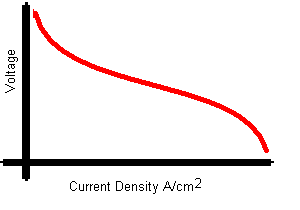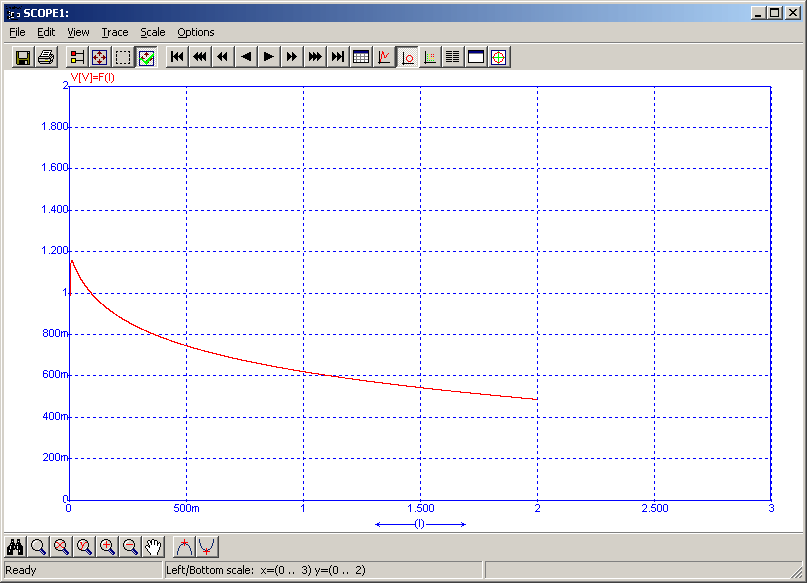• Introduction
• What is in this manual
• What is Caspoc
• User interface
• Introduction
• Starting
• Simulation
• Editing
• Viewing and printing
• Getting Started
• Basic editing
• Simulation in the time domain
• Basic User Interface Topics
• Editing
• Simulation
• Viewing
• Library
• Reports
• Project management
• Circuit and Block Diagram Components
• Introduction
• Cscript and user defined functions
• Component parameters
• Modeling Topics
• Introduction
• Power Electronics
• Semiconductors
• Electrical Machines
• Electrical drives
• Power Systems
• Mechanical Systems
• Thermal Systems
• Magnetic Circuits
• Green Energy
• Coupling to FEM
• Experimenter
• Analog hardware description language
• Embedded C code Export
• Coupling to Spice
• Small Signal Analysis
• Matlab coupling
• Tips and tricks
• Appendices

## Fuel Cell Introduction

There are several models for the Fuel CellAll models are based on the Voltage/Current Density characteristic provided by the manufacturer of the Fuel Cell.The manufacturer provides this data per stack. This data is used in Caspoc and forms the basis of the Fuel Cell model.The above figure shows the characteristic of the level 0 Fuel Cell model. This model is recommended if physical parameters of the Fuel Cell are not known. On the X-axis the current in Amperes is defined. The y-axis is the voltage per stack. By defining the number of stacks in series, the total power produced by the fuel cell can be calculated. The voltage of the fuel cell is depending on the current drawn from the fuel cell as indicated by the graph above.

The Fuel Cell library blocks are selected from the library at Components/Library/GreenEnergy/FuelCell